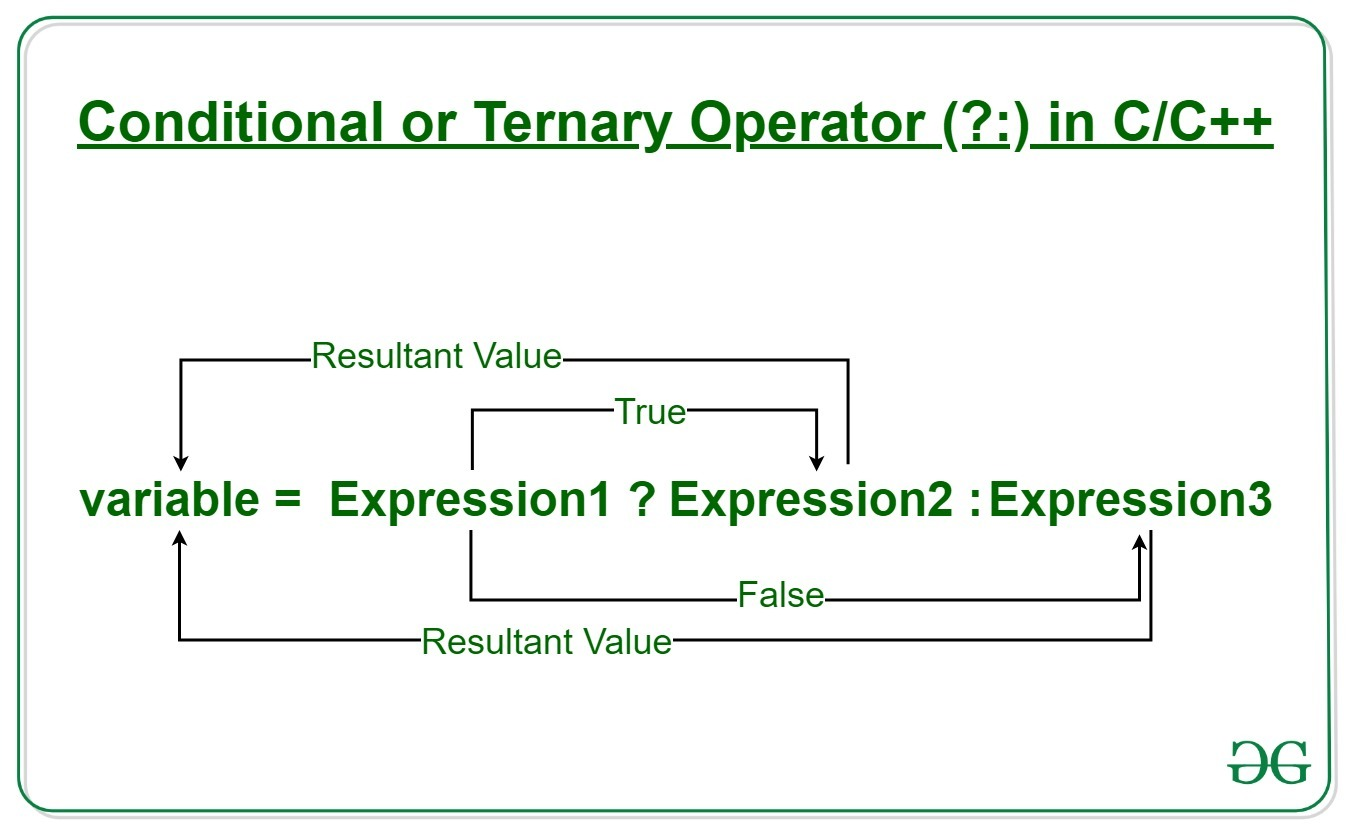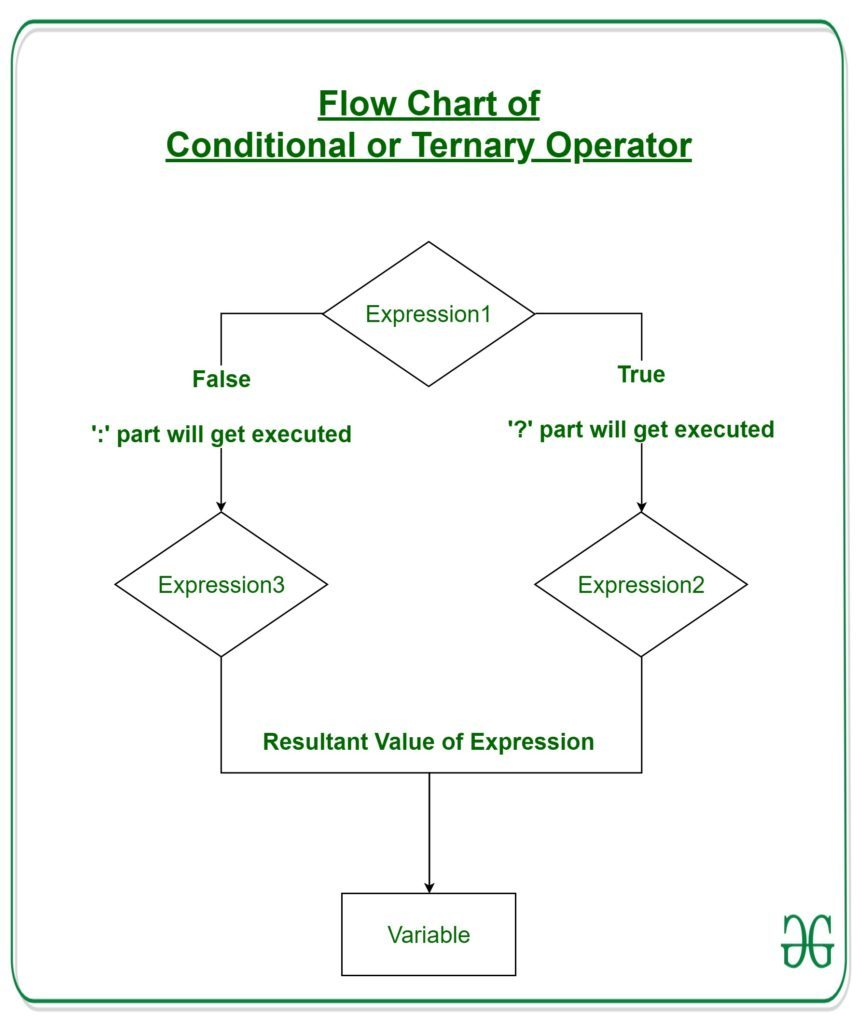# Conditional or Ternary Operator (?:) in C/C++

The conditional operator is kind of similar to the if-else statement as it does follow the same algorithm as of if-else statement but the conditional operator takes less space and helps to write the if-else statements in the shortest way possible.Syntax:
The conditional operator is of the form

`variable = Expression1 ? Expression2 : Expression3`

It can be visualized into if-else statement as:

```if(Expression1)
{
variable = Expression2;
}
else
{
variable = Expression3;
}
```

Since the Conditional Operator ‘?:’ takes three operands to work, hence they are also called ternary operators.

Working:
Here, Expression1 is the condition to be evaluated. If the condition(Expression1) is True then Expression2 will be executed and the result will be returned. Otherwise, if the condition(Expression1) is false then Expression3 will be executed and the result will be returned.Example: Program to Store the greatest of the two Number.

## C

 `// C program to find largest among two ` `// numbers using ternary operator ` ` `  `#include ` ` `  `int` `main() ` `{ ` `    ``// variable declaration ` `    ``int` `n1 = 5, n2 = 10, max; ` ` `  `    ``// Largest among n1 and n2 ` `    ``max = (n1 > n2) ? n1 : n2; ` ` `  `    ``// Print the largest number ` `    ``printf``(``"Largest number between"` `           ``" %d and %d is %d. "``, ` `           ``n1, n2, max); ` ` `  `    ``return` `0; ` `} `

## C++

 `// C++ program to find largest among two ` `// numbers using ternary operator ` ` `  `#include ` `using` `namespace` `std; ` ` `  `int` `main() ` `{ ` `    ``// variable declaration ` `    ``int` `n1 = 5, n2 = 10, max; ` ` `  `    ``// Largest among n1 and n2 ` `    ``max = (n1 > n2) ? n1 : n2; ` ` `  `    ``// Print the largest number ` `    ``cout << ``"Largest number between "` `         ``<< n1 << ``" and "` `         ``<< n2 << ``" is "` `         ``<< max; ` ` `  `    ``return` `0; ` `} `

Output:

```Largest number between 5 and 10 is 10.
```

Attention reader! Don’t stop learning now. Get hold of all the important DSA concepts with the DSA Self Paced Course at a student-friendly price and become industry ready.

My Personal Notes arrow_drop_upCheck out this Author's contributed articles.

If you like GeeksforGeeks and would like to contribute, you can also write an article using contribute.geeksforgeeks.org or mail your article to contribute@geeksforgeeks.org. See your article appearing on the GeeksforGeeks main page and help other Geeks.

Please Improve this article if you find anything incorrect by clicking on the "Improve Article" button below.

Article Tags :
Practice Tags :

7

Please write to us at contribute@geeksforgeeks.org to report any issue with the above content.